Ex 8.3

Chapter 8 Class 8 Algebraic Expressions and Identities
Serial order wise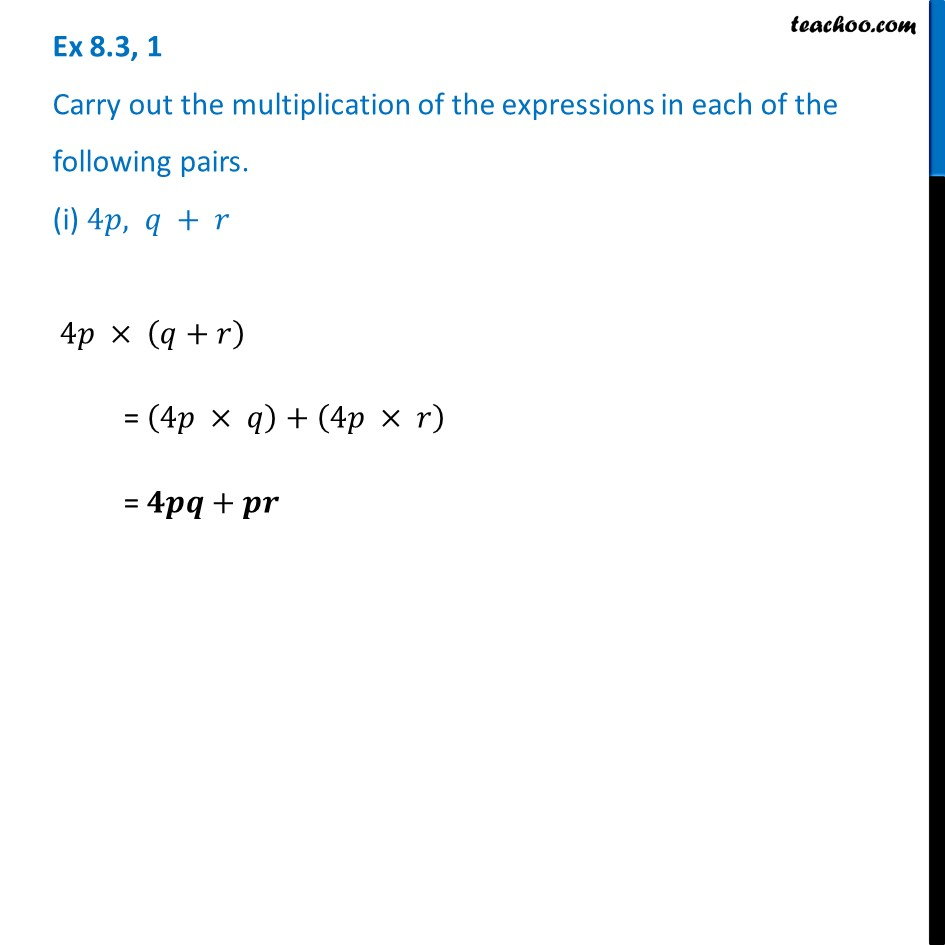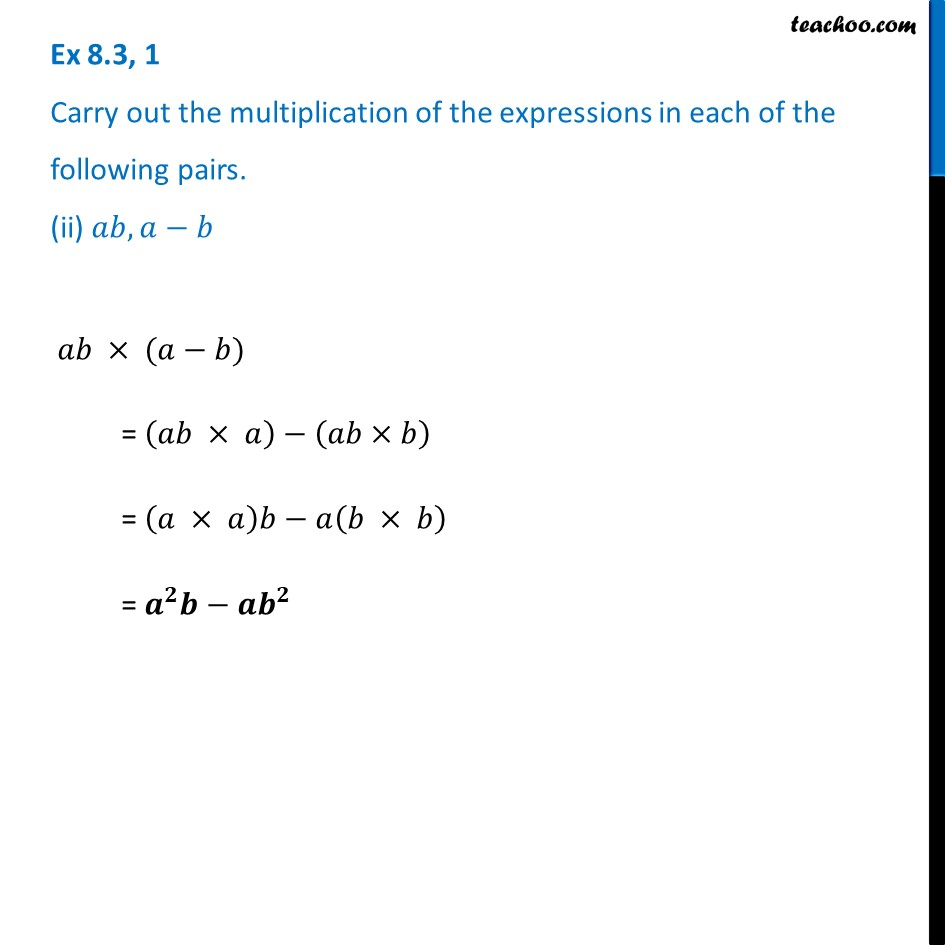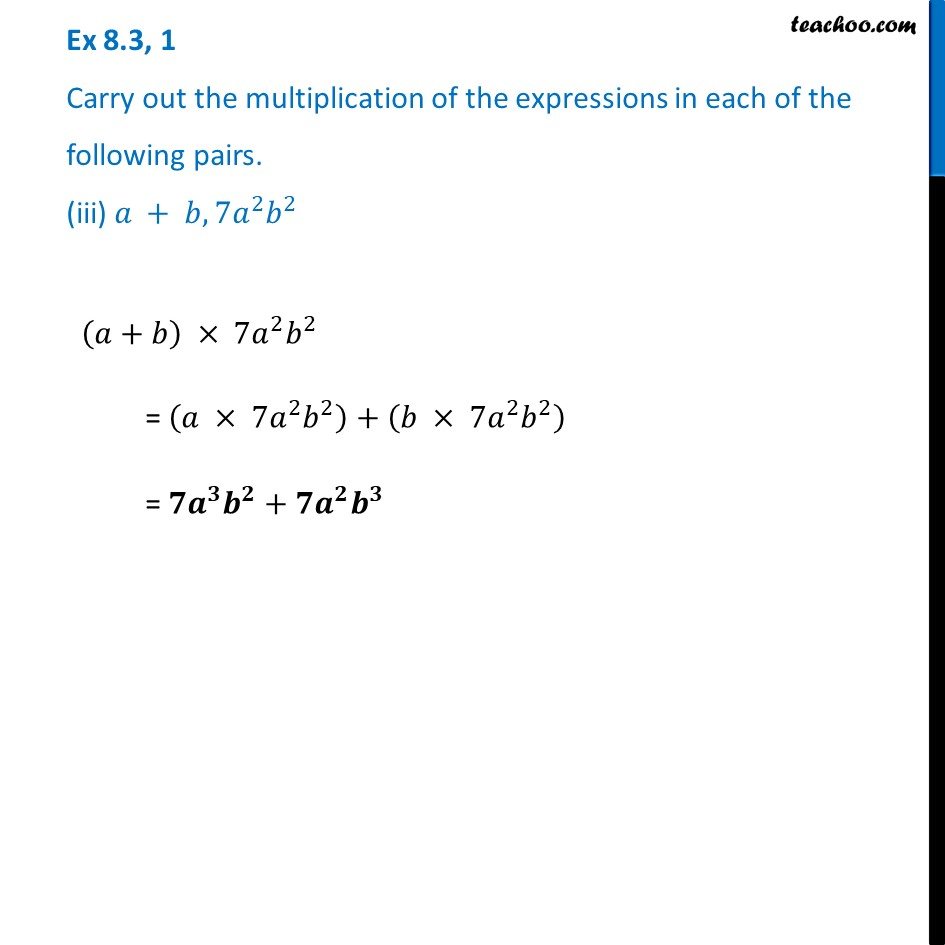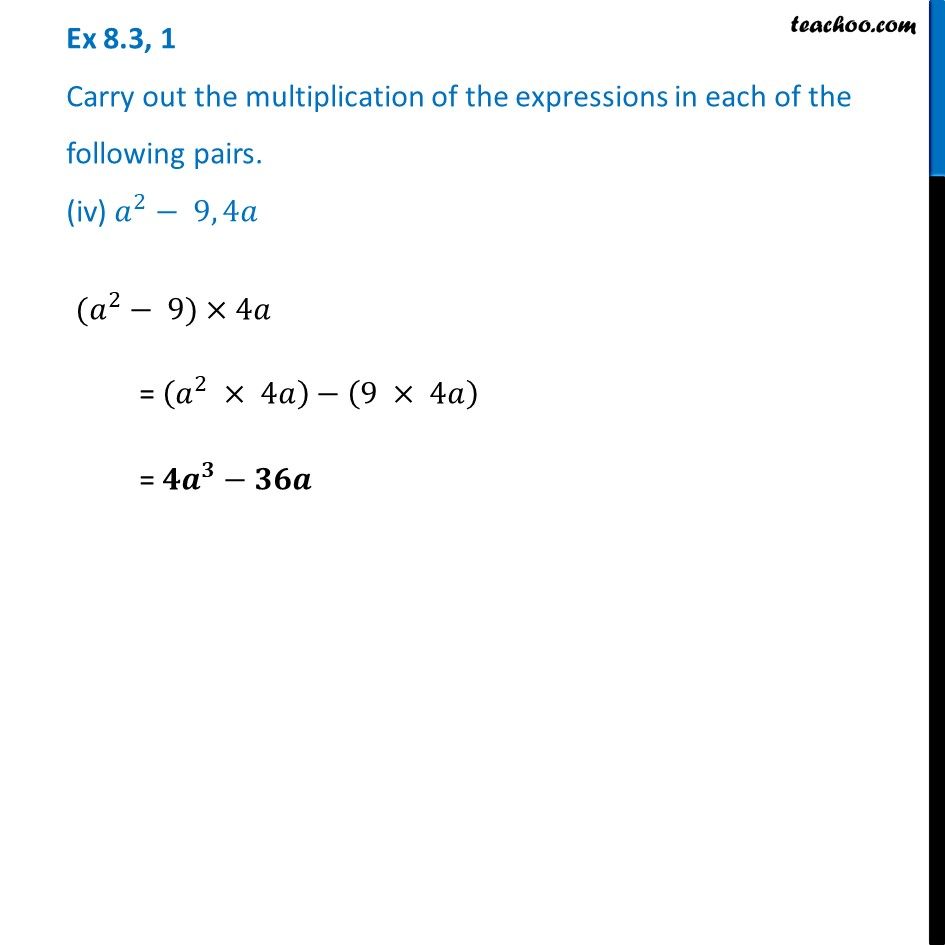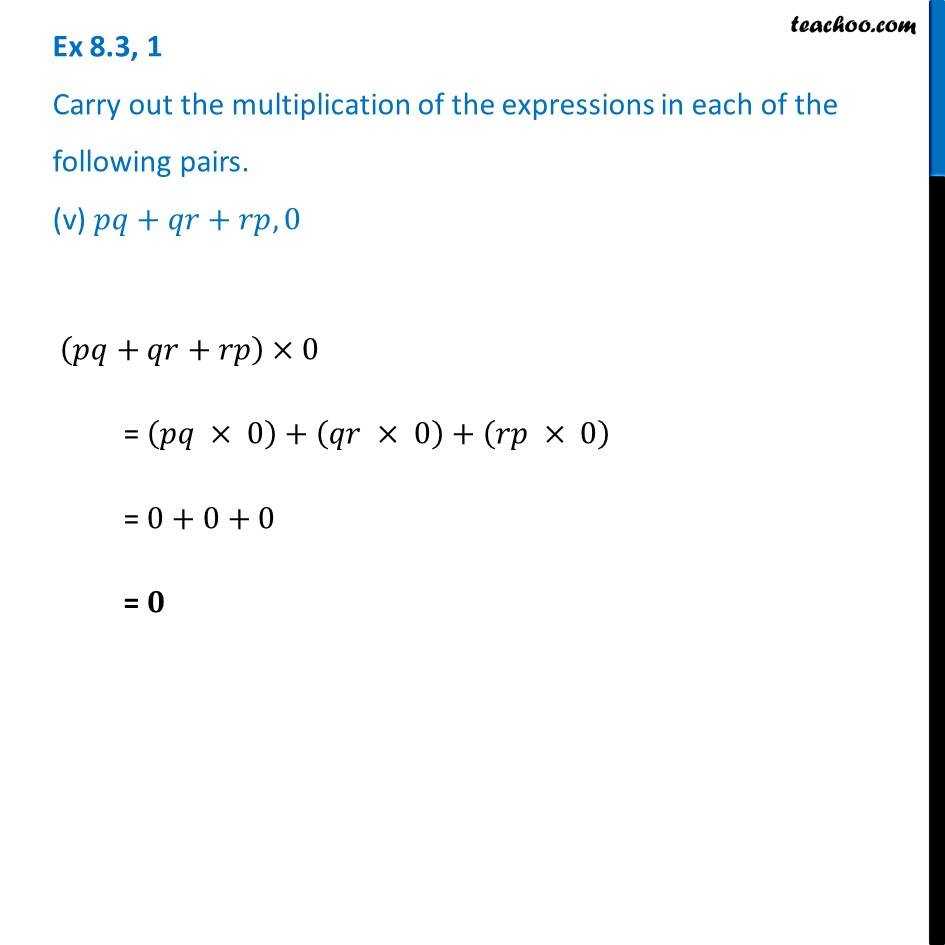Learn in your speed, with individual attention - Teachoo Maths 1-on-1 Class

### Transcript

Ex 8.3, 1 Carry out the multiplication of the expressions in each of the following pairs. (i) 4𝑝, 𝑞 + 𝑟 4𝑝 × (𝑞+𝑟) = (4𝑝 × 𝑞)+(4𝑝 × 𝑟) = 𝟒𝒑𝒒+𝒑𝒓 Ex 8.3, 1 Carry out the multiplication of the expressions in each of the following pairs. (ii) 𝑎𝑏, 𝑎−𝑏 𝑎𝑏 × (𝑎−𝑏) = (𝑎𝑏 × 𝑎)−(𝑎𝑏×𝑏) = (𝑎 × 𝑎)𝑏−𝑎(𝑏 × 𝑏) = 𝒂^𝟐 𝒃−𝒂𝒃^𝟐 Ex 8.3, 1 Carry out the multiplication of the expressions in each of the following pairs. (iii) 𝑎 + 𝑏, 7𝑎^2 𝑏^2 (𝑎+𝑏) × 7𝑎^2 𝑏^2 = (𝑎 × 7𝑎^2 𝑏^2 )+(𝑏 × 7𝑎^2 𝑏^2 ) = 𝟕𝒂^𝟑 𝒃^𝟐+𝟕𝒂^𝟐 𝒃^𝟑 Ex 8.3, 1 Carry out the multiplication of the expressions in each of the following pairs. (iv) 𝑎^2− 9, 4𝑎 (𝑎^2− 9)×4𝑎 = (𝑎^2 × 4𝑎)−(9 × 4𝑎) = 𝟒𝒂^𝟑−𝟑𝟔𝒂 Ex 8.3, 1 Carry out the multiplication of the expressions in each of the following pairs. (v) 𝑝𝑞+𝑞𝑟+𝑟𝑝, 0 (𝑝𝑞+𝑞𝑟+𝑟𝑝)×0 = (𝑝𝑞 × 0)+(𝑞𝑟 × 0)+(𝑟𝑝 × 0) = 0+0+0 = 𝟎